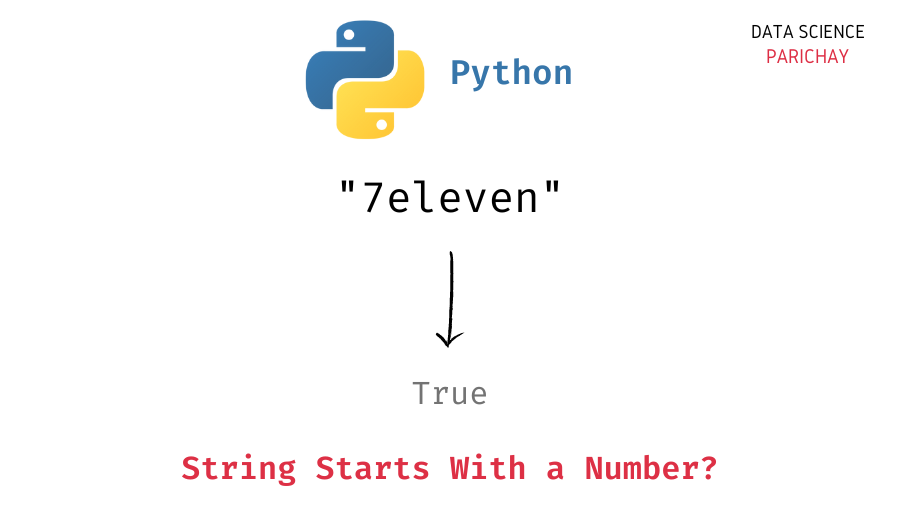# Python – Check If String starts with a Number

In this tutorial, we will look at how to check if a string in Python starts with a number (digit) or not with the help of some examples.

## How to check if a string starts with a numeric character?

To check if a string in Python starts with a number or not, check if the first character in the string is a digit or not using the string `isdigit()` function.

The built-in string `isdigit()` function in Python takes a string and returns `True` if all the characters in the string are numeric characters and there is at least one character in the string. It returns `False` otherwise.

So, use the string `isdigit()` function to check if the first character in the string (character at index `0`) is a digit. The following is the syntax –

```# check if string starts with a number
s.isdigit()```

It returns `True` if the string starts with a numeric character.

## Examples

Let’s now look at some examples. First, we will create some strings that we will use throughout this tutorial.

```# create strings
s1 = "7eleven"
s2 = "apple"
s3 = "\$500"
s4 = ""

# display the strings
print(s1)
print(s2)
print(s3)
print(s4)```

Output:

```7eleven
apple
\$500
```

Here, we created four strings – `s1`, `s2`, `s3` and `s4`. The string `s1` starts with a digit, the string `s2` starts with an alphabet, the string `s3` starts with the special character `'\$'` and the string `s4` is an empty string.

📚 Data Science Programs By Skill Level

Introductory

Intermediate ⭐⭐⭐

🔎 Find Data Science Programs 👨‍💻 111,889 already enrolled

Disclaimer: Data Science Parichay is reader supported. When you purchase a course through a link on this site, we may earn a small commission at no additional cost to you. Earned commissions help support this website and its team of writers.

Let’s now check if the strings `s1`, `s2`, and `s3` start with a digit or not.

```# check if string starts wtih a number
print(s1.isdigit())
print(s2.isdigit())
print(s3.isdigit())```

Output:

```True
False
False```

We get `True` for `s1` as it does start with a digit. We get `False` for `s2` and `s3` as they do not start with a digit.

Now let’s apply the above method to the empty string, `s4`.

```# check if string starts wtih a number
print(s4.isdigit())```

Output:

```---------------------------------------------------------------------------
IndexError                                Traceback (most recent call last)
Input In , in <module>
1 # check if string starts wtih a number
----> 2 print(s4.isdigit())

IndexError: string index out of range```

We get an `IndexError` because we’re trying to access an index that does not exist (an empty string does not have a `0` index).

To avoid the above error, you can first check whether the string is non-empty and then proceed to check if the first character is a digit or not. See the example below –

```# function to check if string starts wtih a digit
def check_str_starts_with_digit(s):
if s and s.isdigit():
return True
else:
return False

# check if string starts wtih a number
print(check_str_starts_with_digit(s4))```

Output:

`False`

We get `False` as the output for the empty string.

You might also be interested in –

•